# Using MONTH and EOMONTH functions in Excel - formula examples

The tutorial explains the nuts and bolts of Excel MONTH and EOMONTH functions. You will find an array of formula examples demonstrating how to extract month from date in Excel, get the first and last day of the month, convert month name to number and more.

In the previous article, we explored a variety of formulas to calculate weekdays. Today, we are going to operate on a bigger time unit and learn the functions that Microsoft Excel provides for months.

In this tutorial, you will learn:

## Excel MONTH function - syntax and uses

Microsoft Excel provides a special MONTH function to extract a month from date, which returns the month number ranging from 1 (January) to 12 (December).

The MONTH function can be used in all versions of Excel 2016 - 2000 and its syntax is as simple as it can possibly be:

MONTH(serial_number)

Where `serial_number` is any valid date of the month you are trying to find.

For the correct work of Excel MONTH formulas, a date should be entered by using the DATE(year, month, day) function. For example, the formula `=MONTH(DATE(2015,3,1))` returns 3 since DATE represents the 1st day of March, 2015.

Formulas like `=MONTH("1-Mar-2015")` also work fine, though problems may occur in more complex scenarios if dates are entered as text.

In practice, instead of specifying a date within the MONTH function, it's more convenient to refer to a cell with a date or supply a date returned by some other function. For example:

`=MONTH(A1)` - returns the month of a date in cell A1.

`=MONTH(TODAY())` - returns the number of the current month.

At first sight, the Excel MONTH function may look plain. But look through the below examples and you will be amazed to know how many useful things it can actually do.

## How to get month number from date in Excel

There are several ways to get month from date in Excel. Which one to choose depends on exactly what result you are trying to achieve.

MONTH function in Excel - get month number from date

This is the most obvious and easiest way to convert date to month in Excel. For example:

• `=MONTH(A2)` - returns the month of a date in cell A2.
• `=MONTH(DATE(2015,4,15))` - returns 4 corresponding to April.
• `=MONTH("15-Apr-2015")` - obviously, returns number 4 too.

TEXT function in Excel - extract month as a text string

An alternative way to get a month number from an Excel date is using the TEXT function:

• `=TEXT(A2, "m")` - returns a month number without a leading zero, as 1 - 12.
• `=TEXT(A2,"mm")` - returns a month number with a leading zero, as 01 - 12.

Please be very careful when using TEXT formulas, because they always return month numbers as text strings. So, if you plan to perform some further calculations or use the returned numbers in other formulas, you'd better stick with the Excel MONTH function.

The following screenshot demonstrates the results returned by all of the above formulas. Please notice the right alignment of numbers returned by the MONTH function (cells C2 and C3) as opposed to left-aligned text values returned by the TEXT functions (cells C4 and C5).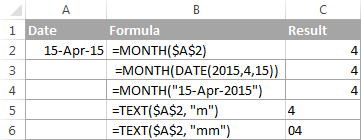## How to extract month name from date in Excel

In case you want to get a month name rather than a number, you use the TEXT function again, but with a different date code:

• `=TEXT(A2, "mmm")` - returns an abbreviated month name, as Jan - Dec.
• `=TEXT(A2,"mmmm")` - returns a full month name, as January - December.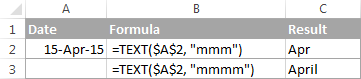If you don't actually want to convert date to month in your Excel worksheet, you are just wish to display a month name only instead of the full date, then you don't want any formulas.

Select a cell(s) with dates, press Ctrl+1 to opent the Format Cells dialog. On the Number tab, select Custom and type either "mmm" or "mmmm" in the Type box to display abbreviated or full month names, respectively. In this case, your entries will remain fully functional Excel dates that you can use in calculations and other formulas. For more details about changing the date format, please see Creating a custom date format in Excel.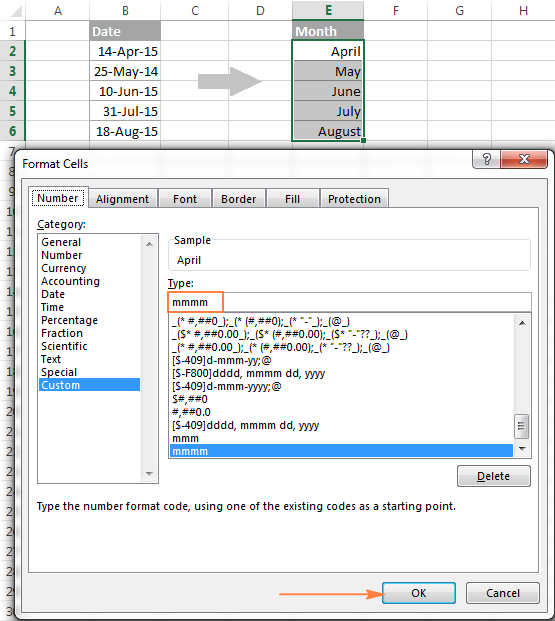## How to convert month number to month name in Excel

Suppose, you have a list of numbers (1 through 12) in your Excel worksheet that you want to convert to month names. To do this, you can use any of the following formulas:

To return an abbreviated month name (Jan - Dec):

`=TEXT(A2*28, "mmm")`

`=TEXT(DATE(2015, A2, 1), "mmm")`

To return a full month name (January - December):

`=TEXT(A2*28, "mmmm")`

`=TEXT(DATE(2015, A2, 1), "mmmm")`

In all of the above formulas, A2 is a cell with a month number. And the only real difference between the formulas is the month codes:

• "mmm" - 3-letter abbreviation of the month, such as Jan - Dec
• "mmmm" - month spelled out completely
• "mmmmm" - the first letter of the month name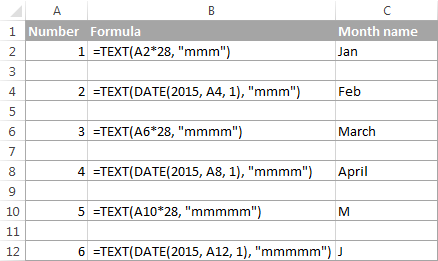### How these formulas work

When used together with month format codes such as "mmm" and "mmmm", Excel considers the number 1 as Day 1 in January 1900. Multiplying 1, 2, 3 etc. by 28, you are getting Days 28, 56, 84, etc. of the year 1900, which are in January, February, March, etc. The format code "mmm" or "mmmm" displays only the month name.

## How to convert month name to number in Excel

There are two Excel functions that can help you convert month names to numbers - DATEVALUE and MONTH. Excel's DATEVALUE function converts a date stored as text to a serial number that Microsoft Excel recognizes as a date. And then, the MONTH function extracts a month number from that date.

The complete formula is as follows:

`=MONTH(DATEVALUE(A2 & "1"))`

Where A2 in a cell containing the month name you want to turn into a number (&"1" is added for the DATEVALUE function to understand it's a date).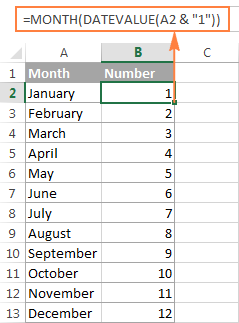## How to get the last day of month in Excel (EOMONTH function)

The EOMONTH function in Excel is used to return the last day of the month based on the specified start date. It has the following arguments, both of which are required:

EOMONTH(start_date, months)
• Start_date - the starting date or a reference to a cell with the start date.
• Months - the number of months before or after the start date. Use a positive value for future dates and negative value for past dates.

Here are a few EOMONTH formula examples:

`=EOMONTH(A2, 1)` - returns the last day of the month, one month after the date in cell A2.

`=EOMONTH(A2, -1)` - returns the last day of the month, one month before the date in cell A2.

Instead of a cell reference, you can hardcode a date in your EOMONTH formula. For example, both of the below formulas return the last day in April.

`=EOMONTH("15-Apr-2015", 0)`

`=EOMONTH(DATE(2015,4,15), 0)`

To return the last day of the current month, you use the TODAY() function in the first argument of your EOMONTH formula so that today's date is taken as the start date. And, you put 0 in the `months` argument because you don't want to change the month either way.

`=EOMONTH(TODAY(), 0)`

Note. Since the Excel EOMONTH function returns the serial number representing the date, you have to apply the date format to a cell(s) with your formulas. Please see How to change date format in Excel for the detailed steps.

And here are the results returned by the Excel EOMONTH formulas discussed above: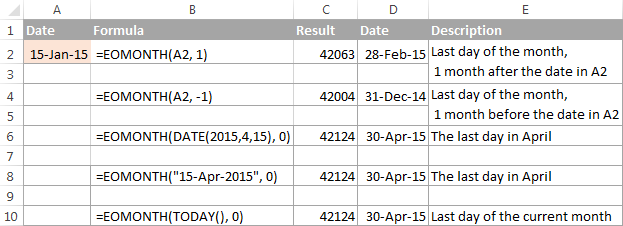If you want to calculate how many days are left till the end of the current month, you simply subtract the date returned by TODAY() from the date returned by EOMONTH and apply the General format to a cell:

`=EOMONTH(TODAY(), 0)-TODAY()`

## How to find the first day of month in Excel

As you already know, Microsoft Excel provides just one function to return the last day of the month (EOMONTH). When it comes to the first day of the month, there is more than one way to get it.

#### Example 1. Get the 1st day of month by the month number

If you have the month number, then use a simple DATE formula like this:

=DATE(year, month number, 1)

For example, =DATE(2015, 4, 1) will return 1-Apr-15.

If your numbers are located in a certain column, say in column A, you can add a cell reference directly in the formula:

`=DATE(2015, B2, 1)`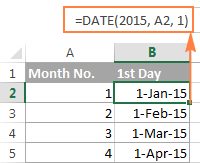#### Example 2. Get the 1st day of month from a date

If you want to calculate the first day of the month based on a date, you can use the Excel DATE function again, but this time you will also need the MONTH function to extract the month number:

=DATE(year, MONTH(cell with the date), 1)

For example, the following formula will return the first day of the month based on the date in cell A2:

`=DATE(2015,MONTH(A2),1)`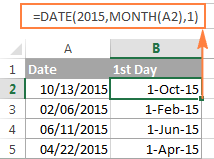#### Example 3. Find the first day of month based on the current date

When your calculations are based on today's date, use a liaison of the Excel EOMONTH and TODAY functions:

`=EOMONTH(TODAY(),0) +1` - returns the 1st day of the following month.

As you remember, we already used a similar EOMONTH formula to get the last day of the current month. And now, you simply add 1 to that formula to get the first day of the next month.

In a similar manner, you can get the first day of the previous and current month:

`=EOMONTH(TODAY(),-2) +1` - returns the 1st day of the previous month.

`=EOMONTH(TODAY(),-1) +1` - returns the 1st day of the current month.

You could also use the Excel DATE function to handle this task, though the formulas would be a bit longer. For example, guess what the following formula does?

`=DATE(YEAR(TODAY()), MONTH(TODAY()), 1)`

Yep, it returns the first day of the current month.

And how do you force it to return the first day of the following or previous month? Hands down :) Just add or subtract 1 to/from the current month:

To return the first day of the following month:

`=DATE(YEAR(TODAY()), MONTH(TODAY())+1, 1)`

To return the first day of the previous month:

`=DATE(YEAR(TODAY()), MONTH(TODAY())-1, 1)`

## How to calculate the number of days in a month

In Microsoft Excel, there exist a variety of functions to work with dates and times. However, it lacks a function for calculating the number of days in a given month. So, we'll need to make up for that omission with our own formulas.

#### Example 1. To get the number of days based on the month number

If you know the month number, the following DAY / DATE formula will return the number of days in that month:

=DAY(DATE(year, month number + 1, 1) -1)

In the above formula, the DATE function returns the first day of the following month, from which you subtract 1 to get the last day of the month you want. And then, the DAY function converts the date to a day number.

For example, the following formula returns the number of days in April (the 4th month in the year).

`=DAY(DATE(2015, 4 +1, 1) -1)`

#### Example 2. To get the number of days in a month based on date

If you don't know a month number but have any date within that month, you can use the YEAR and MONTH functions to extract the year and month number from the date. Just embed them in the DAY / DATE formula discussed in the above example, and it will tell you how many days a given month contains:

`=DAY(DATE(YEAR(A2), MONTH(A2) +1, 1) -1)`

Where A2 is cell with a date.

Alternatively, you can use a much simpler DAY / EOMONTH formula. As you remember, the Excel EOMONTH function returns the last day of the month, so you don't need any additional calculations:

`=DAY(EOMONTH(A1, 0))`

The following screenshot demonstrates the results returned by all of the formulas, and as you see they are identical: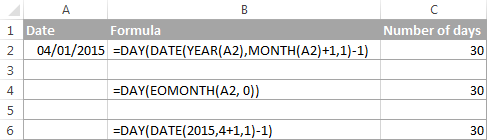## How to sum data by month in Excel

In a large table with lots of data, you may often need to get a sum of values for a given month. And this might be a problem if the data was not entered in chronological order.

The easiest solution is to add a helper column with a simple Excel MONTH formula that will convert dates to month numbers. Say, if your dates are in column A, you use =MONTH(A2).

And now, write down a list of numbers (from 1 to 12, or only those month numbers that are of interest to you) in an empty column, and sum values for each month using a SUMIF formula similar to this:

`=SUMIF(C2:C15, E2, B2:B15)`

Where E2 is the month number.

The following screenshot shows the result of the calculations: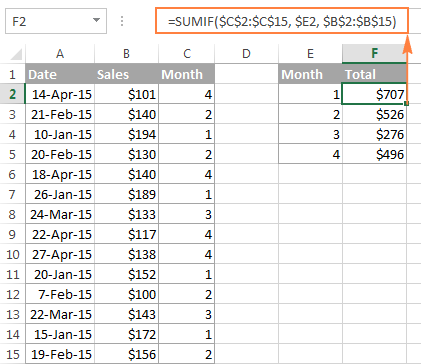If you'd rather not add a helper column to your Excel sheet, no problem, you can do without it. A bit more trickier SUMPRODUCT function will work a treat:

`=SUMPRODUCT((MONTH(\$A\$2:\$A\$15)=\$E2) * (\$B\$2:\$B\$15))`

Where column A contains dates, column B contains the values to sum and E2 is the month number.

Note. Please keep in mind that both of the above solutions add up all values for a given month regardless of the year. So, if your Excel worksheet contains data for several years, all of it will be summed.

## How to conditionally format dates based on month

Now that you know how to use the Excel MONTH and EOMONTH functions to perform various calculations in your worksheets, you may take a step further and improve the visual presentation. For this, we are going to use the capabilities of Excel conditional formatting for dates.

In addition to the examples provided in the above mentioned article, now I will show you how you can quickly highlight all cells or entire rows related to a certain month.

#### Example 1. Highlight dates within the current month

In the table from the previous example, suppose you want to highlight all rows with the current month dates.

First off, you extract the month numbers from dates in column A using the simplest =MONTH(\$A2) formula. And then, you compare those numbers with the current month returned by =MONTH(TODAY()). As a result, you have the following formula which returns TRUE if the months' numbers match, FALSE otherwise:

`=MONTH(\$A2)=MONTH(TODAY())`

Create an Excel conditional formatting rule based on this formula, and your result may resemble the screenshot below (the article was written in April, so all April dates are highlighted).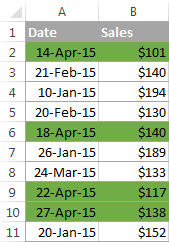#### Example 2. Highlighting dates by month and day

And here's another challenge. Suppose you want to highlight the major holidays in your worksheet regardless of the year. Let's say Christmas and New Year days. How would you approach this task?

Simply use the Excel DAY function to extract the day of the month (1 - 31) and the MONTH function to get the month number, and then check if the DAY is equal to either 25 or 31, and if the MONTH is equal to 12:

`=AND(OR(DAY(\$A2)=25, DAY(\$A2)=31), MONTH(A2)=12)`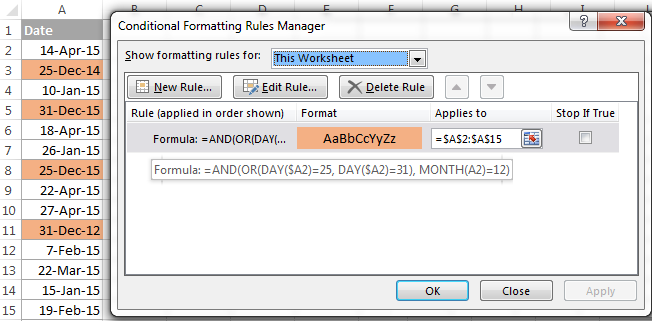This is how the MONTH function in Excel works. It appears to be far more versatile than it looks, huh?

In a couple of the next posts, we are going to calculate weeks and years and hopefully you will learn a few more useful tricks. If you are interested in smaller time units, please check out the previous parts of our Excel Dates series (you will find the links below). I thank you for reading and hope to see you next week!

## You may also be interested in

1. I am looking to create a table to calculate and monitor battery life. The user can input in cell C7 the expected battery life of a product in months (24 months). The surrounding cells are percentages of that and as such the outputs are in a # of months and # days.

For example, I expect when no LEDs are on to get the battery life of 24 months.
I expect when all LEDs are on that my expected battery life should be 1/10th of that, meaning 2 months and 12 days (rounded).

2. Hi Alex

Please I want to change the format of short month to long month on another column, which formular do I use?

E.g I want Jan to return January but on another Column

3. Hi
Hope all will be fine.
When I apply date format to text "10-06-2023", it converts it to 06-10-2023 or 06-Oct-2023. But I need the result 10-Jun-2023. How to sort it out?

• Thnx for the reply. But I have done everything to sort it out but in vain. Then I tried CSV and import process and finally resolved.

4. Hi - I'm looking to calculate % of days that fall in each quarter based on a start and end date, counting the start date as a day in the quarter and in the total. For example: I have a start date of 3/26 and end date of 4/9. That's 6 days in Q1 (40%) and 9 days (60%) in Q2. Is there a formula I can use to output number of days in Q1 and number of days in Q2? Is there a formula I can use to calculate the % of total days that fall in a given quarter?

5. Hi! I need to create a formula that inserts the number of the month (1-12) into a cell if the date falls between the 1st and the 15th of the month. If the date falls between the 16th and EOM, I need to put the number of the month (1-12) into another cell.

Cells in Column A are used to calculate income that is received between the 1st and 15th.
Cells in Column B are used to calculate income that is received between the 16th and EOM.

These cells will be used in a formula to determine where to add income amounts on a different sheet within the workbook.

• Hi! Use the DAY function to determine the day, and use the MONTH function to get the month number according to the condition.

=IF(DAY(D1)<16,MONTH(D1),"")

6. used =TEXT(A5; "mmm") where A5 was a date formated as 2022-06-19 returned either 00 or =TEXT(A5; "mmm")

I am presumably missing something obvious!

• Hi! I don't know what local date settings are used in your Windows. I assume that the date is written as text. To get the date, try these recommendations: How to convert text to date and number to date in Excel.
You can extract the day, month and year from the text and create a date using the DATE function.

=DATE(LEFT(A2,SEARCH("-",A2)-1),MID(A2,SEARCH("-",A2)+1,SEARCH("-",A2,SEARCH("-",A2)+1)-SEARCH("-",A2)-1),RIGHT(A2,LEN(A2)-SEARCH("-",A2,SEARCH("-",A2,1)+1)))

7. Hi I need some help please.

I need to get extract data from a table of every working day in month, but only that which falls on the last day of the month. The data set contains multiple years worth of data so need it specifically for each month of each year.
Is this possible?

Data looks like this:

Date Value of transactions Number Of transactions Largest transaction
04/01/2021 47 96 6
05/01/2021 35 46 12

So I would need the value of transactions for the last working day of every month for 2021, 2022, etc

Thanks

• Hi! To extract the row with the last date of a month, if any date of that month is written in F1, you can use the INDEX MATCH formula:

=INDEX(A1:D100,MATCH(EOMONTH(F1,0),A1:A100,1),)

8. Hi,

I have 2 sheet first one includes the following details:

employee ID 12346 and leave balance up to end of the year (33)

second sheet in includes the following details: employee approved leave history

Emp ID leave start leave end
12346 20-Jan-23 20-Jan-23
12346 01-Mar-23 03-Mar-23

which formula will help to know the how may leave been take per month

for example i what the sheet to give me

Employee ID
number of leave taken in Jan
number of leave taken in Mar

best Regards

• please i need an urgent support if you can please to the above concern

9. Hello Expert.
I appreciate the good work you are doing.
I have a problem to solve. I have a table to monitor the treatment of patients in the continuum of care. There is a particular column with the Date of the next clinic appointment with varying dates. I want a formula that will return all dates with the current month in another sheet and it will copy all the columns with information in them for the clients that will come to the clinic in the month.

• Thanks for the quick response. I found the link you directed me to useful, however, the filter function only works with Excel 2021 upward. My Excel is an old version and is there any other way to go about it using formula.

• Hello! In your Excel, you can try using an Excel filter to get the records you want and then manually copy them to a new worksheet. Also take a look at the Pivot Table.

10. Hello Alex

Thanks for this amazing forum and Q&A. I need a quick help on the below. I have below Set of Data and I wanted to get the last Month Name for a particular App Id. As an example for App Id "123456" I am expecting Jan-24 as output and similarly for AppId "987654" expecting Feb-24 as the output. Can you guide how can I get it.

App Id Month Name
123456 Jul-23
123456 Sep-23
123456 Aug-23
123456 Jan-24
123456 Dec-23
987654 Sep-23
987654 Oct-23
987654 Feb-24

• In cell B1, write this formula to split the text into ID and date. After that, you can copy this formula down along the column.

=TEXTSPLIT(A1," ")*1

Hello! In cell D1, write the formula and copy down along the column.

=TEXT(MAXIFS(\$S\$1:\$S\$8,\$R\$1:\$R\$8,B1),"mmm-yy")

• Hello Alex

Thank you for your quick response. It helped to certain extent but not meeting the expected result. In below table "Result" columns showing the value after applying the below formula. Expected column shows the value I am expecting. As you can see for App Id 123456, value should be Jan-24 and AppId: 987654 Value should be Feb-24. Similarly there will be more App Id and for which I am expecting to get MMM-YY as the result. Its basically expecting for each App ID what is the last month and Yr (MMM-YY) in the month column. Can you help.

=TEXT(MAXIFS(\$B\$2:\$B\$10,\$A\$2:\$A\$10,C1),"mmm-yy")

App Id Month Result Expected
123456 Jul-23 Jan-00 Jan-24
123456 Sep-23 Jan-00 Jan-24
123456 Aug-23 Jan-00 Jan-24
123456 Jan-24 Jan-00 Jan-24
123456 Dec-23 Jan-00 Jan-24
987654 Jan-24 Jan-00 Feb-24
987654 Sep-23 Jan-00 Feb-24
987654 Oct-23 Jan-00 Feb-24
987654 Feb-24 Jan-00 Feb-24

11. Hi Alexander Trifuntov.

Scenario: Company months overlap between two months; i.e. June sales are recorded from the 26th May until the 25th June.
Excel will recognize sales from the 26th May until 31st May as May sales.

Question: Which formula can be used to look at the 26th May until 25th June as "June"?

This way, company stakeholders will not argue with the data presented.

• Hello! If I understand your task correctly, the following formula should work for you:

=MONTH(IF(DAY(A1)>25,EOMONTH(A1,1),A1))

• Hi!
Thank you very much - it worked like a charm! :)

12. Hi Alexander, I have a question that I don't think has come up when I read your answers. I have the following text in cell A2: 'Credit for April 2023'. Now I am trying to convert the month, April in this example, to a date in 2023 in cell B2; and preferably the last date of that month (YYYY-MM-DD 2023-04-30). I have succeeded in converting the name of the month to number 4 (the 4th month of the year), but I can't figure out how to change the month number into the last day of the month. Maybe I am doing this all wrong, not sure, but I hope you can you help me with this?

• Hello! To get the last day of the current month, use the EOMONTH function. Try this formula:

=EOMONTH(DATE(RIGHT(A2,4), MONTH(1&CHOOSECOLS(TEXTSPLIT(A2," "),3)), 1),0)

13. Hi sir,

I have a spreadsheet (exported by Jira) for which every entry has a start date in the format as 15/JApr/23 7:51 AM. To calculate how many days have passed since that start date i input as end date (today's date) in format 21/06/23 and by using the Days360 function i get the age. The problem is that i have to manually change the exported format (of the exported start day) to dd/mm/yy by using find and replace where Jan ->01, Feb->02 etc. or else the Days360 formula will not work.

Could you think of any alternative to convert the date?

• Hi! To replace part of the text, you can use the REPLACE function. For example:

=REPLACE(A1,4,4, VLOOKUP(MID(A1,5,3),{"JAN",1;"FEB",2;"MAR",3;"APR",4;"May",5;"JUN",6;"JUL",7;"AUG",8;"Sep",9;"OCT",10;"NOV",11;"DEC",12},2,0))

• Thank you, sir!

14. Hi! I have a column with dates. I have another column where I want to display seasonal limits: 2.8 from June to October and 5.7 from November to May. How do I write a formula that displays the correct limit for any year? Thank you!

• Hi! Unfortunately, this information is not enough to understand what you need.

I have column A indicate: 10 in weeks. How do I capture the 10 (weeks) into number in months?

16. Hi! I need to be able to find a future date on a set day of the month.

Example:
Training Date: 2/27/23
Launch Date: 4 months forward
Deadline day (Same day every month): 25th

I need a formula to auto calculate 4 months forward from the training date, and then the next 25th.
So in this example, the formula should return 7/25/23.

If the training date was: 2/23/23, 4 months forward, on the 25th, should return 6/25/23.

Does anyone know the correct date formula for this?

• I think I've got it!

B5=Training Date
B9=Deadline day (same date every month/shows a number: 1, 5, 10, 15, etc).
=IF(DAY(B5)>B9,EOMONTH(B5,4)+B9, EOMONTH(B5,3)+B9)

Does anyone see an issue with this working?

17. Hi,

I have a spreadsheet with 11/30/23 in a date-defined field in A1. In E1 there is an IF statement: =IF(MONTH(TODAY()=MONTH(A1)),"Yes","No")

The result is "Yes" even though today's month is 5 and doesn't equal the month in A1 which is 11.

Thanks so much!

• Hi!
Try this formula:

=IF(MONTH(TODAY())=MONTH(A1),"Yes","No")

Also check cell A1. Perhaps there is text and not the date.

18. Dear Team, I want to present the last 3 months of data , the month should be change dynamically in future. tell the formula

• Hello! I can't see your data, so I can't advise you on any formula. But you can get the date of the last day of the month 3 months before the current month using the EOMONTH function.

=EOMONTH(TODAY(),-3)

If you need to subtract exactly 3 months from the current date, use this instruction: How to add and subtract dates in Excel.

19. Hi Alex, sorry if theres a real simple solution to this but I can't stop excel from resetting month count once it exceeds 12.

Eg I'm using =month(I5-G5-X5) to work out the months between the date range. In this example there's actually 14 months but excel is reverting to 2.

Any ideas?

20. Greetings Alex, I am searching for a formula solution that will enable me to populate data, by month in a two dimensional array. I am tracking income adjustments over a 12 month calendar year. For example if a salary adjustments are made sometime during April - December, I need to populate the new (adjusted) salary through the rest of the year, while at the same time maintaining the pre-adjustment salaries. In my attempts at a correct formula, pervious salaries (static and adjusted) will take on the new (adjusted) values. I think I can preserve salaries, previous to any adjustments by limiting salary adjustments to the remaining months in the year. I hope this makes sense.

• Hello! Your task is not completely clear to me. To ensure clear understanding of your task, can you provide an instance of the input data and the desired result you are aiming for?

• Still trying to resolve this issue, Alex. For example, I am trying to adjust income that occurs this month. I can use this formula where O2 is the cell containing the number of the month and the index value is the income from the previous month. E9 contains the adjusted amount (if any) for this month. K15 contains the original monthly income and does not change.
=IF(O2=5,IF(INDEX(D19:O30,12,4)E9,IF(E9=K15,K15,IF(E9K15,E9,))))

This formula works OK for the current month. On the other hand, I can't use this formula for other than the current month, i.e. I want to preserve the adjusted income amounts (if any for the previous month). Your suggestions are always welcome.

• I made a mistake in the above formula. Here is the corrected formula. Sorry for any confusion.

=IF(O2=5,IF(INDEX(D19:O30,12,4)E9,E9,IF(E9=K15,K15,E9)))

• Hi! I can't check the formula as I don't have your data. But I assume you can change the number 5 to a different month number.

• OK, Here is some data. Assume that K15 contains the monthly income of \$7000.00 (populated at the beginning of the year [January]. K15 will not change during the year. E9 also is populated with the same monthly income at the beginning of the year as K15. E9 can change as monthly income goes up or down. In May E9 has a value of 8,000. monthly income which means that there was a 1,000. increase in previous months Jan-Ap. Both K15 and E9 are outside of the table. The table contains 12 columns, one for each month, and a cell for monthly income in each column.

My approach has been to compare the previous month's total income (April) to E9 (8000). if a change (in this case, 1,000) then use E9 for May. IF E9 is = to K15 (7000) then I use K15 for May. If E9 (8000) to K15 then use E9 for May. I am using a formula, in the monthly income cell, for each month in the table. As above this formula works for May: =IF(O2=5,IF(INDEX(D19:O30,12,4)E9,E9,IF(E9=K15,K15,E9))) .

I can not use this formula for months Jan - April because, for example, O2 (month # = 5 in May) 4 in April column and will return a "False" instead of preserving the original income (7000) for April. Sorry for this long post. Maybe my solution of preserving static and adjusted income through out the year is not the right one.

• Hi! Unfortunately, without seeing your data, I can't understand what you want to do. But I don't think you can use one cell with a formula for all months.

• Hi Alex, Here is some Demo Data from a partial table.
Summary Monthly Expense Reports

Jan-23 Feb-23 Mar-23 Apr-23 May-23 Jun-23
Miscellaneous Expenses \$49.00 \$135.00 \$300.00 \$0.00 \$0.00 \$0.00
Grocery \$887.00 \$435.00 \$400.00 \$0.00 \$0.00 \$0.00
Car Fuel & Maintenance \$116.00 \$162.00 \$0.00 \$0.00 \$0.00 \$0.00
Dental \$0.00 \$2,600.00 \$1,500.00 \$0.00 \$0.00 \$0.00
Vitamins \$77.00 \$0.00 \$0.00 \$0.00 \$0.00 \$0.00
Health & Well Being \$109.00 \$121.00 \$0.00 \$0.00 \$0.00 \$0.00
Fixed Monthly Expenses \$4,278.00 \$4,278.00\$4,278.00 \$4,278.00 \$4,278.00 \$4,278.00
Monthly Fees w Annual Due Dates\$172.00 \$172.00 \$172.00 \$172.00 \$172.00 \$172.00
Member/Card Fees: Ann Due Date\$21.00 \$21.00 \$21.00 \$21.00 \$21.00 \$21.00

Total Net Monthly Income \$7,000.00 \$7,000.00\$7,000.00 *FALSE\$7,142.86** \$7,142.86
Total Actual Monthly Expenses \$5,709.00\$7,924.00\$6,671.00 \$4,471.00\$4,471.00 \$4,471.00
Monthly Balances \$1,291.00 \$924.00 \$329.00 \$4,471.00 \$2,671.86 \$2,671.86
*Formula in the cell for April's Total Net Monthly Income: =IF(O2=4,IF(INDEX(D19:O30,12,3)E9,E9,IF(E9=K15,K15,IF(E9K15,E9,))))
** Formula in the cell for May's Total Net Monthly Income: =IF(O2=5,IF(INDEX(D19:O30,12,4)E9,E9,IF(E9=K15,K15,E9)))
Looking for a formula that I can use for both the current month's Total Net Monthly Income and all the other non-current months.

• Unfortunately, this information did not help me understand what you want to do. We apologize, but we cannot offer assistance beyond the scope of this blog. The solution to your query requires a more comprehensive approach and cannot be addressed through a simple formula. However, if you have a specific question about a function or formula, feel free to ask, and we will do our best to assist you.

21. I want to get no of completed months between two dates i.e. 01-06-1990 to 30-06-1990 or 31-05-1990 to 30-06-1990 (completed one months ),
but when date is 02-06-1990 to 30-06-1990 or 01-06-1990 to 29-06-1990, this is not completed months between two dates ,
please guide which excel formula will be suitable for my requirement

22. Thanks for the excellent detail and generous set of examples! I was able to quickly find exactly what I need to convert a number (1-12) to a month name.

• In a CSV file, I receive a text value that contains a date & time value in this format: MM/DD/YY HH:MM
I want to convert it to a month number and full month name in this format: MM -
Using the function that you provided above to convert a month number to a month name, I created this formula:
=LEFT(B2,2) & " - " & TEXT(LEFT(B2,2)*28, "mmmm")

LEFT(B2,2) - Provides a two-digit month number based on the original date & time value
TEXT(LEFT(B2,2)*28, "mmmm") - Provides the full month name based on the month number

Examples:
Original Date & Time Value My Formula Returns
03/31/23 09:16pm 03 - March
04/03/23 03:09am 04 - April

• Hi!
Create a date with the desired month number using the DATE function and apply a custom date format using the TEXT function to get the month name.

=TEXT(DATE(2023,A1,1),"Mmmm")

23. Hi, I need to write a function of condition if my date exceeds 15 months of some stated date (noth munt, excatd date/day) that it should be marked as "expired".

For example started day is 25.8.2022 and from TODAY function is that date passed 15 months.

• You are a life saver! Thank you, it works :)

24. =COUNTIFS(Jobsites!\$S\$2:\$S\$5000,\$A5,Jobsites!\$F\$2:\$F\$5000,">="&(DATE(\$B\$2,(MONTH(DATEVALUE(\$B\$1&"1"))),1)),Jobsites!\$F\$2:\$F\$5000,"<="&(DATE(\$B\$2,(MONTH(DATEVALUE(\$B\$1&"1"))),EOMONTH(DATE(\$B\$2,(MONTH(DATEVALUE(\$B\$1&"1"))),1),0))))

This is my formula. The date I am referencing is April 2022 (month is written). If I change the EOMonth formula portion to 30, it will calculate correctly. However, as it is now, it is not calculating correctly. Please help!

• Hi!
I cannot check your formula because it contains unique references to your data. I assume you need to correct formula of the month -

MONTH(DATEVALUE("1"&\$B\$1))

25. I'm trying to get a cell to say the name of the month based off a date in another cell. The cell does not contain a year so the formula =TEXT(D56,"MMMM") is not working. Any ideas? Thank you!

• What is written in cell D56? If the date is written there, the formula works.

• I was given a spreadsheet with birthdays but there is no year. D56 shows 05/26 for May 26th.

• Hi!
You don't write the date, you have text. Extract the month number and create the date using the DATE function.

=TEXT(DATE(2023,LEFT(D56,2),1),"MMMM")

• Thank you!! You are the best!!

26. I am trying to convert a date to number of months. Can that be done in Excel?

• Hi! You can convert the date to the number of months using the MONTH function, which is described in detail above.

27. Current formula used to count REMAINING number of months and the way I formulated is creating a negative digit but is the numeric I need.. How to get rid of the negative?

=MONTH(DATEVALUE(cell & "1"))-12

example: November is giving me -1, January is giving me -11. Would like these to be whole numbers

• Figured it out on my own moving the 12 to the front like a nitwit! Thanks anyway. Hope this helps others

=12-(MONTH(DATEVALUE(cell &"1")))

November is giving me 1 now :)

28. What is the importance of "*28" in the formula =TEXT(A2*28, "mmm")?

Although I got my result right after pacing *28 in my formula, I would like to understand why my formula, was retuning "Jan" instead of "Feb" for a date of 28-02-2023 in cell B2
The formula that returned a wrong result is =TEXT(MONTH(B2), "mmm")
The formula that returned a correct result is =TEXT((MONTH(B2))*28, "mmm")

29. Hi. I am trying to use the =MONTH(TODAY()) function to show the month as a number in a cell, but I would like the month number 1 to start as April (tax year start). Is this possible to do?

Regards
Gareth

• Hi!
Try to use IF function:

=IF(MONTH(TODAY())<4,MONTH(TODAY())+9,MONTH(TODAY()))

30. Dear experts,
I am trying to obtain a date value to a cell depending on the date of another cell.
Practically:
Cell A1 is a date
Cell A2 result should be:
if date of A1 + 8 days is less or equal to 15 of the month of A1, then A2 should show 15th of the month of A1; otherwise it should be date of A1 + 8 days.
I tried this formula =IF((A1+8<=15);15;(A1+8)) but it is not working.
I think that I am unable to say that "15"' is the date related to the month of A1.

• Hello!
If I understand your task correctly, use the DATE function to get the desired date

=IF(A1+8<=DATE(YEAR(A1),MONTH(A1),15),DATE(YEAR(A1),MONTH(A1),15),A1+8)

• Thank you very much Alexander, yes you did indeed understand me correctly.

31. hi

I have a problem with this formulas

=IF(B2="","",IF(EOMONTH(\$J\$1,0)>=B2+1,B2+1,"")

how can I correct it ?

thx

• Hi!

=IF(B2="","",IF(EOMONTH(\$J\$1,0)>=B2+1,B2+1,""))

32. Dear Experts,

How I can get the return of last month in same year based on month and year?

I use this formula:

=TEXT(EOMONTH('Stock Report fv'!B2,-1),"mmmm-yy")

To get August -22 but it returns August -23

Stock Report fv'!B2 = September-22

Regards

• Hi!
Check the cell value and cell format. I think September-22 is 22 September 2023.

• Hi Alexandre

Thank you for kind and quick reply. In fact what I am trying to do :
1. I have a stock ledger with many entries from September 2022 till date
2. I want to create the stock report with columns "opening balance" , " In" , "Out", "Closing Balance"
3. For Opening balance I used Sumifs formula and one of the criteria I put "<="&EOMONTH(\$B\$2,-1) - sum in & out from the inventory for all previous months

4. I behaves like that for one item the 1st entry was done in september (40 Pieces), until now the we issues 22 pieces the stock balanec should be 18 as of today. I have Cell (B2) with name of months with year with combo box to select. When I select september -22 openning balance is 18 (should be zero) total received is 40 (which is right) - closing balance is Okay.

5. The formula I used for opening balance is

=IF(ISBLANK(\$A5),"",IF(\$B\$2="All",0,SUMIFS('Stock Ledger'!\$M\$2:\$M\$10000,'Stock Ledger'!\$A\$2:\$A\$10000,'Stock Report fv'!\$A5,'Stock Ledger'!\$B\$2:\$B\$10000,"<="&EOMONTH(\$B\$2,-1))-SUMIFS('Stock Ledger'!\$N\$2:\$N\$10000,'Stock Ledger'!\$A\$2:\$A\$10000,'Stock Report fv'!\$A5,'Stock Ledger'!\$B\$2:\$B\$10000,"<="&EOMONTH(\$B\$2,-1))))

A 5 is unique value

• Hi!
I can't check the formula that contains unique references to your workbook worksheets, sorry. If you select september -22 in the combo box, then Excel will automatically convert this text to the date 01-09-2022

• Hi Alexandre, Thank you for your reply and support - it was data type mismatch. The combo was populated through Text () - converted the list into date and now it works
Thanks

33. In case it helps anyone else:
I ended up making helper rows, and then the following formula works

=IF(SUMPRODUCT(--(Sales!\$B:\$B=\$A3),--(Sales!\$I:\$I=J\$1),--(Sales!\$H:\$H=J\$2))=0,"","X")

--(Sales!\$B:\$B=\$A3) <- this is my purchaser code
--(Sales!\$I:\$I=J\$1) <- this is the year in the helper row
--(Sales!\$H:\$H=J\$2) <-this is the month in the helper row

If all the conditions aren't met, it equals 0, so I wrapped it in the IF function to change the zeros to blanks, and just give me an X when there was a result.

34. Hello,

I have a sheet "Development" in which I show how much overtime our employees have made last month, current year. I wanna choose between Jan-Dec.

Range("G1").Value = "Month"

Range("G2").FormulaR1C1 = _
"=TEXT(DATE(1,MONTH(TODAY())-1,1), ""MM.YYYY"")"

But the problem is that only the year doesn't show up when I run the makro. It's just displays:
"12.YYYY"

But I want something like that: "12.2022" or "December 2022"
From our HR Tool I imported all data automatically into a excel table.

• Hi!
In January, MONTH(TODAY())-1 returns 0.
Use MONTH(EOMONTH(TODAY(),-1))

35. I have a sheet with orders on it, column B has the purchaser code, and column G has the date. Several of our purchasers buy sporadically, so I'd like to be able to visually see which months a purchaser has bought something without having to filter by each purchaser.

I was hoping to make a section of a second sheet with the purchaser code in column A, and then the next columns contain the possible months/years (November 2021, December 2021, January 2022, etc). I've tried using the month and year functions, but I'm getting value errors ("A value used in the formula is of the wrong data type"). I suspect it's because I'm having the month function look at an entire column, but I need the entire column evaluated. I've checked the formats too. I'm not sure what is going wrong, and perhaps this is a silly way to be trying this anyway, but I need a scatter plot type visual, and I can't get the excel scatter chart to work right, because it views each time the purchaser code shows up as a separate thing.

=IF(AND(MONTH(Sales!G:G)=J\$2,YEAR(Sales!G:G)=\$J\$1,Sales!B:B=\$A3),X,"")

Sales!G:G is the list of dates (formatted as date)
Sales!B:B has the purchaser code (as does A3)
J2 and J1 have the month and year numbers respectively, since it wasn't working with the date there. I've formatted J1 and J2 as both number and general, and neither seems to matter

The MONTH(Sales!G:G)=J\$2 and YEAR(Sales!G:G)=\$J\$1 portions were modeled after the "How to sum data by month" sumproducts section.

I also tried the following formula, and it gets the value error as well
=SUMPRODUCT((MONTH(Sales!G:G)=J\$2),(1))

This also errors:
=SUMPRODUCT((MONTH(Sales!G:G)=J\$2),(Sales!I:I)) - Sales!I:I has more numerical data

and this errors:
=SUMPRODUCT((Sales!G:G)*(Sales!I:I))

but this does not error:
=SUMPRODUCT((Sales!G:G),(Sales!I:I))
(so side note, the * in sum product may or may not still work)

• Hello!
To find matches within a range of values, use the MATCH function. Here is an example formula:

=IF(ISERROR(MATCH(J2,MONTH(Sheet1!G1:G20),0) +MATCH(J1,YEAR(Sheet1!G1:G20),0) +MATCH(A3,Sheet1!A1:A20,0)),"","X")

Hope this is what you need.

• Thanks. Unfortunately, something isn't working quite right. It seems to just be looking to see if each are on the list, instead of looking to see if all 3 conditions show up on the same line.

These are the dates for 2021, with a letter to represent the purchaser code
A 11/21/21
B 12/13/21
B 12/13/21
B 12/13/21
B 12/13/21
C 12/16/21
A 12/21/21
D 12/21/21
E 12/22/21
F 12/23/21

I want to get data that looks something like this
2021
11 12
A X X
B X
C X
D X
E X
F X

• Here's a second try at what I'd like the data to look like (it let me paste in a tab space, but removed it when I hit send)

2021
11 12
A X X
B X
C X
D X
E X
F X

• Urg
Last try (ignore the dashes if they come through)
-------2021
------11---12
A-----X-----X
B------------X
C------------X
D-----------X
E------------X
F------------X

• Hello!
Try this formula:

=IF(ISERROR(MATCH(J2,MONTH(Sheet1!G1:G20),0) * MATCH(J1,YEAR(Sheet1!G1:G20),0) * MATCH(A3,Sheet1!A1:A20,0)),"","X")